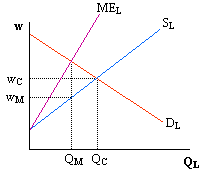Barry Haworth
University of Louisville
Department of Economics
Economics 301

### Monopsony

In output markets with only one seller, there is monopoly. When there is only one buyer in a factor market, we have monopsony. A key difference between monopsonistic and competitive factor markets is how total costs change with the purchase of additional factors.

For example, in a competitive labor market, firms may purchase as many laborers as needed at the market wage because each firm's labor supply curve is horizontal. Because a monopsonist is the only buyer of labor in a particular labor market, the monopsonist faces the entire (positively sloped) market labor supply curve. The difference is clearly important when considering the marginal cost of hiring each additional worker.

When facing a positively sloped market supply curve, the firm's total costs change with the hiring of additional workers as follows:

DTC = wDL + (Dw)L

If we divide both sides of the equation by DL and then rearrange, we have:

DTC/DL = w + (Dw/DL)L

This equation is refered to as the marginal expenditure of labor. Note that the marginal expenditure of labor (or marginal cost of hiring additional workers) is greater than the wage here. Therefore, the marginal expenditure curve is steeper than the market labor supply curve.

In addition, because the monopsonist hires a quantity of labor that coincides with where the marginal cost of hiring each additional worker equals the marginal benefit of each additional worker, the firm hires at the point where the firm's demand for labor equals the marginal expenditure for labor. This is illustrated on the graph below.On the graph, the marginal expenditure equals the firm's demand for labor at the quantity QM. To get this quantity of workers to supply labor, the firm must pay a wage that comes from the labor supply curve. On the graph, that wage is wM .

Contrary to the monopsonistic market, hiring in a competitive labor market occurs where the demand for labor equals the supply of labor. To provide a comparison with a similar monopsonistic labor market, the competitive market equilibrium values are included in the graph as well (QC and wC). Note that a monopsonist would hire less labor and pay a lower wage than what would result in competitive labor market with similar demand and supply conditions.